# Methods of Valuation – Corporate and Management Accounting MCQ

Going through the Methods of Valuation – Corporate and Management Accounting CS Executive MCQ Questions with Answers you can quickly revise the concepts.

## Methods of Valuation – Corporate and Management Accounting MCQs

Question 1.
Security Analysis is a process of estimating ……… for individual securities.
(A) Return and risk
(B) Risk and correlation
(C) Correlation and co-efficient
(D) Return and co-efficient
(A) Return and risk

Question 2.
Return from listed security is in two forms -……….
(A) One is interest and second is capital appreciation in price.
(B) One is stock split and second is dividend.
(C) One is interest and second is dividend.
(D) One is dividend and second is capital appreciation in price.
(D) One is dividend and second is capital appreciation in price.

Question 3.
Which of the following is correct formula to calculate returns of listed security?
(A) [(P1 – P0) + D] ÷ P0  ×100
(B) [(P1 – P0) + D] ÷ P1 × 100
(C) [(P1 – P0) – D] ÷ P0 × 100
(D) [(P1 – P0) + D (1 -t)] -r P0 × 100
(A) [(P1 – P0) + D] ÷ P0  ×100

Question 4.
The market price of a bond depend on –
(A) The coupon rate and terms of the indenture
(B) The coupon rate and maturity date
(C) The terms of the indenture, and maturity date
(D) The coupon rate, terms of the indenture, and maturity date
(D) The coupon rate, terms of the indenture, and maturity date

Question 5.
Liquidity risk:
(A) is risk investments bankers face
(B) is lower for small companies
(C) is risk associated with secondary market transactions
(D) increases whenever interest rates increases
(C) is risk associated with secondary market transactions

Question 6.
A risk associated with project and way considered by well diversified stockholder is classified as –
(A) Expected risk
(B) Beta risk
(C) Industry risk
(D) Returning risk
(B) Beta risk

Question 7.
An attempt to make correction by adjusting historical beta to make it closer to an average beta is classified as –

Question 8.
A corporate bond is a corporation’s write undertaking that it will refund a specific amount of money plus –
(B) Interest
(C) Nothing
(D) Security
(B) Interest

Question 9.
The common stock of a company must provide a higher expected return than the debt of the same company because
(A) There is less demand for stock than for bonds.
(B) There is greater demand for stock than for bonds.
(C) There is more systematic risk involved for the common stock.
(D) There is a market premium required for bonds.
(C) There is more systematic risk involved for the common stock.

Question 10.
A main difference among real and nominal interest proceeds is that –
(A) Real returns adjust for inflation and nominal returns do not
(B) Real returns use actual cash flows and nominal use expected cash flows
(C) Real interest adjusts for commissions and nominal returns do not
(D) Real returns show highest possible return and nominal returns show lowest possible return
(A) Real returns adjust for inflation and nominal returns do not

Question 11.
The beta of the market portfolio is:
(A) -1.0
(B) 0
(C) 1.0
(D) 0.5
(C) 1.0

Question 12.
Non-systematic risk is furthermore identified as –
(A) No diversifLable risk
(B) Market risk
(C) Random risk
(D) Company specific risk
(D) Company specific risk

Question 13.
Beta is measure of –
(A) Non-diversifiable risk
(B) Diversifiable risk
(C) Total risk
(D) Covariance
(A) Non-diversifiable risk

Question 14.
Investors should be agreeing to invest in riskier investments merely –
(A) If return is short
(B) If there are no safe alternatives except for holding cash
(C) If expected return is adequate for risk level
(D) If there are true speculators
(C) If expected return is adequate for risk level

Question 15.
A beta of 1.15 for a security would indicate that –
(A) Security is trading 15% higher than market index.
(B) Security is 15% riskier than index/ market
(C) Security is 15% less risky than index/ market.
(D) Security and market has covariance of 0.15.
(B) Security is 15% riskier than index/ market

Question 16.
A beta of 0.8 for a security would indicate that –
(A) Security is 20% riskier than index/ market.
(B) Security is 80% less risky than index/ market.
(C) Security is 20% less risky than index/ market.
(D) Security is 80% riskier than index/ market.
(C) Security is 20% less risky than index/ market.

Question 17.
Which of the following is correct formula to calculate beta (β)?(D) All of the above
(D) All of the above

Question 18.
Capital Asset Pricing Model (CAPM) provides the link between –
(A) Return and total risk
(B) Risk and covariance
(C) Return and non-diversifiable risk
(D) Return and diversifiable risk
(C) Return and non-diversifiable risk

Question 19.
Which of the following investment advice will you provide to your client investor if CAPM Return < Expected return?
(A) Sell
(B) Hold
(D) Short

Question 20.
Which of the following investment advice will you provide to your client investor if CAPM Return > Expected return?
(A) Sell
(C) Hold
(D) None of the above
(A) Sell

Question 21.
If expected return is more than required return as per CAPM, then –
(A) Security is overvalued and hence can be bought
(B) Security is correctly priced and hence should be hold
(C) Security is undervalued and hence can be sold
(D) Security is undervalued and hence can be bought
(C) Security is undervalued and hence can be sold

Question 22.
The Security Market Line (SML) is a line drawn on a chart that serves as a graphical representation of the Capital Asset Pricing Model, which shows different levels of………. of various marketable securities plotted against the expected return.
(A) Systematic risk
(B) Unsystematic risk
(C) Total risk
(D) Free risk
(A) Systematic risk

Question 23.
Market risk is also called:
(A) Systematic risk and unique risk.
(B) Unique risk & non diversihable risk.
(C) Systematic risk & diversihable risk.
(D) Non diversihable risk & systematic risk.
(D) Non diversihable risk & systematic risk.

Question 24.
Which of the following investment advice will you provide to your client investor if CAPM Return = Expected return?
(A) Sell
(C) Hold
(D) Put
(C) Hold

Question 25.
If required return as per CAPM is more them expected return, then –
(A) Security is undervalued and can be sold
(B) Security is correctly priced and hence should be hold
(C) Security is overvalued and hence can be bought
(D) Security is undervalued and hence can be bought
(A) Security is undervalued and can be sold

Question 26.
According to the CAPM, overpriced securities have:
(A) Negative alphas
(B) Positive alphas
(C) Zero betas
(D) Zero alphas
(A) Negative alphas

Question 27.
The beta of the risk-free asset is:
(A) 0
(B) 0.5
(C) 2.0
(D) 1.0
(A) 0

Question 28.
Capital asset pricing theory asserts that portfolio returns are best explained by:
(A) Economic factors
(B) Systematic risk
(C) Specihc risk
(D) Diversihcation
(B) Systematic risk

Question 29.
Alpha is an indicator of the extent to which the –
(A) Actual return of a security deviates from those predicated by market experts.
(B) Actual return of a security deviates from those predicated by derivative market.
(C) Actual return of a security deviates from those predicated by its beta value.
(D) Actual return of a security deviates from those predicated by its probable distribution value.
(C) Actual return of a security deviates from those predicated by its beta value.

Question 30.
Alpha is denoted by symbol –
(A) A
(B) ¥
(C) α
(D) ∆
(C) α

Question 31.
Negative alpha value indicates that –
(A) Expected return is less than required return as per CAPM and hence security is overvalued. Such security should be sold.
(B) Expected return is less than required return as per CAPM and hence security is undervalued. Such security should be bought.
(C) Expected return is more than required return as per CAPM and hence security is undervalued. Such security should be bought.
(D) Expected return is equal to required return as per CAPM and hence security is correctly valued. Such security should be hold.
(A) Expected return is less than required return as per CAPM and hence security is overvalued. Such security should be sold.

Question 32.
Systematic Risk is –
(A) Uncontrollable
(B) Controllable
(C) Avoidable
(D) Voidable
(A) Uncontrollable

Question 33.
Positive alpha value indicates that –
(A) Expected return is less than required return as per CAPM and hence security is overvalued. Such security should be sold.
(B) Expected return is more than required return as per CAPM and hence security is undervalued. Such security should be bought.
(C) Expected return is equal to required return as per CAPM and hence security is correctly valued. Such security should be hold.
(D) Expected return is more than required return as per CAPM and hence security is overvalued. Such security should be sold.
(B) Expected return is more than required return as per CAPM and hence security is undervalued. Such security should be bought.

Question 34.
Systematic risk = ?
(A) Beta X SD of market
(B) Total risk – SD of market
(C) Total risk – Beta
(D) Beta 4- SD of market
(A) Beta X SD of market

Question 35.
……….. is also called specific risk.
(A) Systematic risk
(B) Unsystematic risk
(C) Covariance
(D) Coefficient
(B) Unsystematic risk

Question 36.
In contrast to the capital asset pricing model, arbitrage pricing theory:
(A) Uses risk premiums based on micro variables.
(B) Requires normally distributed security returns.
(C) Has fewer restrictive assumptions.
(D) Specifies the number and identities of specific factors that determine expected returns.
(C) Has fewer restrictive assumptions.

Question 37.
The APT is an equilibrium model developed by:
(A) Stephen Ross and Richard Roll
(B) Stephen Ross
(C) Richard Roll
(D) Harry Markowitz
(B) Stephen Ross

Question 38.
Which of the following is NOT an assumption of the APT?
(A) Investors prefer more wealth to less.
(B) Security returns are generated by a linear factor model.
(C) The return-generating process can have more than one common factor.
(D) Investors are risk averse.
(D) Investors are risk averse.

Question 39.
Which of the following statements is correct?
(A) The CAPM is considered to be less restrictive than the APT.
(B) Both the CAPM and the APT are built on the same assumptions.
(C) Both the CAPM and the APT state that there is a linear relation between risk and expected return.
(D) Both the CAPM and the APT predict that all investors will hold the same market portfolio.
(C) Both the CAPM and the APT state that there is a linear relation between risk and expected return.

Question 40.
Which of the following statements about the APT is false?
(A) The major disadvantage of the APT is that it fails to tell us what the common factors are.
(B) The APT requires that securities that provide the same payoffs must sell at the same price.
(C) The APT is considered to be less restrictive than the CAPM.
(D) Most empirical studies show that only one factor is important in the APT.
(D) Most empirical studies show that only one factor is important in the APT.

Question 41.
Which of the following statements is true according to the theory of arbitrage?
(A) Rational investors will arbitrage in a manner consistent with their risk tolerance.
(B) Negative alpha stocks cannot be arbitraged.
(C) Low-beta stocks are consistently overpriced.
(D) Positive alpha stocks will quickly disappear.
(D) Positive alpha stocks will quickly disappear.

Question 42.
Which of the following is an assumption of the APT?
(A) Investors follow the mean-variance rule.
(B) All investors hold the market portfolio.
(C) Investors are risk averse.
(D) Short sales are allowed.
(D) Short sales are allowed.

Question 43.
Following details are available for two securities: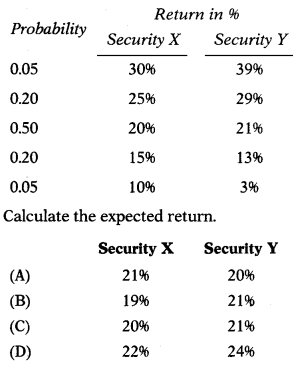(C)
X: (0.05 × 30) + (0.20 × 25) + (0.50 × 20) + (0.20 × 15) + (0.05 × 10) = 20%
Y: (0.05 × 39) + (0.20 × 29) + (0.50 × 21) + (0.20 × 13) + (0.05 × 3) = 21%

Question 44.
Suppose risk free rate is 4% and X is 2.5%. If an investor takes 13% risk, he can expect a return of –
(A) 32.5%
(B) 52%
(C) 10%
(D) 36.5%
(D) 36.5%
Risk free rate is 4% and X is 2.5%. Thus, for every 1% increase in the risk, the investor can expect 2.5% above risk-free return. Thus, if an investor takes 13% risk, he can expect a return of 36.5%
(13 × 2.5)+ 4.

Question 45.
Yogesh invest ₹ 1,25,000 in shares of BABA Ltd., a listed company. At the end of period investment value is ₹ 1,32,000. He gets dividend of ₹ 8,000. Return from investment is –
(A) 11%
(B) 12%
(C) 13%
(D) 14%
(B) 12%
[(1,32,000 – 1,25,000) + 8,000]/1,25,000 × 100 = 12%

Question 46.
Actual return of GK Ltd. for last four year is 20%, 14%, 17% and 18%. GKLtd.has beta of 1.15. Return on market portfolio is 15%. Risk free rate of return is 6%. Compute Alpha value and decide whether to hold, buy or to sell the security?
(A) Alpha value is +0.8 and hence it is advised to sell the security.
(B) Alpha value is -0.9 and hence it is advised to buy the security.
(C) Alpha value is -0.8 and hence it is advised to short sell the security.
(D) Alpha value is +0.9 and hence it is advised to buy the security.
(D) Alpha value is +0.9 and hence it is advised to buy the security.
ER = Rf + β (Rm– Rf)
= 6+1.15(15-6)
=16.35%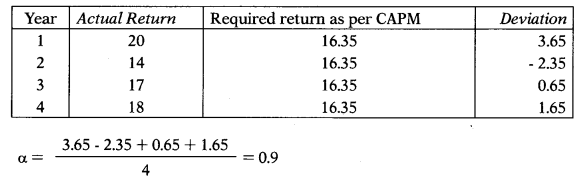Question 47.
Return of last 5 years of listed security is 16.2%, 19.8%, 18%, 15% & 21%. Five years ago price of the security was 120 per share. What is its average return?
(A) 18%
(B) 19%
(C) 20%
(D) 21%
(A) 18%

Question 48.
Return of last 5 years of listed security is 16.2%, 19.8%, 18%, 15% & 21%. Five years ago price of the security was 120 per share.What is its holding period return?
(A) 135.55%
(B) 128.58%
(C) 145.64%
(D) 154.45%
(B) 128.58%
120 × 1.162 × 1.198 × 1.18 × 1.15 × 1.21 = 274.29
(274.29 – 120)/120 × 100 = 128.58%

Question 49.
Dividend for last 4 years of Tara Ltd. was ₹ 7, ₹ 5, ₹ 12.8 & ₹ 10 and market price was ₹ 120, ₹ 80, ₹ 130 & ₹ 150 respectively. What is the average return of last 3 years considering capital gain and dividend?
(A) 19.28%
(B) 14.48%
(C) 10.84%
(D) 16.65%
(A) 19.28%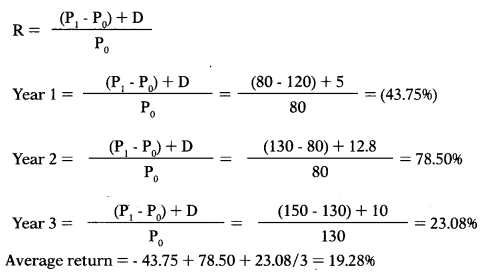Question 50.
Return on XM Ltd. shares has a standard deviation of 23%, as against the standard deviation of the market at 19%. Correlation co-efficient between market and stock of XM Ltd. is 0.8. Compute the systematic risk of XM Ltd.’s shares.
(A) 96.84%
(B) 18.4%
(C) 25.78%
(D) 64.85%
(B) 18.4%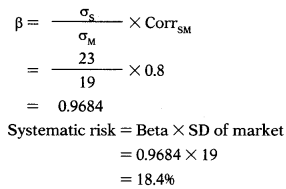Question 51.
Return on Lucky Ltd. shares has a standard deviation of 20%, as against the standard deviation of the market at 15%. Correlation co-efficient between market and stock of XM Ltd. is 0.9. Compute the unsystematic risk of Lucky Ltd.’s shares.
(A) 5%
(B) 2%
(C) 18%
(D) 20%
(B) 2%Question 52.
You are analyzing the beta for ABC Ltd. and have divided the Company into four broad business groups, with market values and betas for each group.Estimate the beta for ABC Ltd. as a company.
(A) 1.275
(B) 1.825
(C) 1.645
(D) 0.895
(A) 1.275Question 53.
Pavan has invested in four securities. Amount invested and beta of each security is as follows: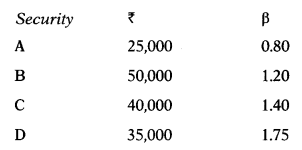Compute portfolio beta.
(A) 1.524
(B) 1.874
(C) 0.789
(D) 1.315
(D) 1.315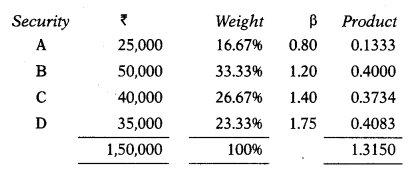Question 54.
ABC Ltd beta is 1.45. Rate of market return is 16%. Rate of return on government securities is 8%. What is the expected return as per Capital Asset Pricing Model? If the risk premium on the market goes up by 2.5% points, what would be revised expected return on this stock?
(A) 16.9%; 32.32%
(B) 18.7%; 24.28%
(C) 19.6%; 23.23%
(D) 19.6%; 16.7%
(C) 19.6%; 23.23%
ER=Rf+p(Rm-Rf)
= 8 + 1.45 (16 – 8)
= 19.6%
Revised expected return if the risk premium on the market goes up by 2.5%:
ER = Rf + p (Rm – Rf)
Note: Risk Premium = (Rm – Rf)
= 8+1.45 (10.5) Revised risk premium = 8 + 2.5 = 10.5 = 23.23%

Question 55.
A financial consultant has gathered following facts for H Ltd.
Systematic risk of the firm is 1.4.
182 days Treasury bill yield is 8%
Expected yield on market portfolio is 13%. Calculate expected return based on capital asset pricing model (CAPM).
(A) 18%
(B) 17%
(C) 16%
(D) 15%
(D) 15%
ER=Rf+p(Rm-Rf)
= 8 + 1.4 (13 – 8)
= 15%

Question 56.
Calculate the required return on the security from the following information:
Standard deviation 2.5%
Market standard deviation 2.0%
Risk free rate of return 13%
Expected rate of return on 15%
market portfolio
Correlation coefficient of 0.8
portfolio with the market
(A) 16%
(B) 15%
(C) 14%
(D) 12%
(B) 15%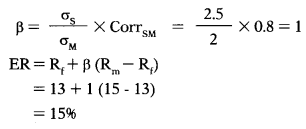Question 57.
Expected return of Security A is 22% while that of Security B is 24%. Beta of Security A is 0.86 while that of Security B is 1.24. What is risk free rate?
(A) 14.74%
(B) 17.47%
(C) 14.71%
(D) 14.00%
(B) 17.47%Question 58.
The expected return and beta of three securities is as follows: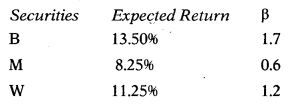If the risk free rate of return is 6.75% and the expected return on market portfolio is 10.5%, which of the above securities under or correctly valued?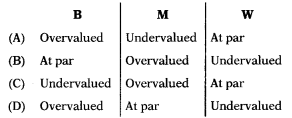(A) Overvalued
(B) At par
(C) Undervalued
(D) Overvalued
(C) Undervalued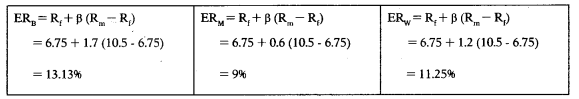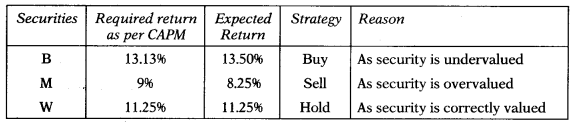Question 59.
Actual return of T Ltd. for last four year is as follows:T Ltd. has beta of 1.15. Return on market portfolio is 11.2%. Risk free rate of return is 4%. Compute Alpha value and decide whether to hold, buy or to sell the security.
(A) Alpha value is positive Le. 0.4 and hence security is overvalued. Such security should be sold.
(B) Alpha value is positive ie. 0.4 and hence security is undervalued. Such security should be bought.
(C) Alpha value is negative Le. – 0.2 and hence security is overvalued. Such security should be sold.
(D) Alpha value is negative Le. – 0.2 and hence security is undervalued. Such security should be bought.
(B) Alpha value is positive ie. 0.4 and hence security is undervalued. Such security should be bought.Alpha value is positive i.e. 0.4 and hence security is undervalued. Such security should be bought.

Question 60.
Following details are made available to you for particular security:
Beta of security : 0.5
Expected return on portfolio : 15%
Risk free rate of return : 0.06
In another security has an expected rate of return of 18%, what would be its beta?
(A) 1.222
(B) 1.111
(C) 1.333
(D) 1.444
(C) 1.333
ER=Rf+p(Rm-Rf)
= 6 + 0.5 (15 – 6)
= 10.5%
Computation of beta for other security:
ER=Rf+p(Rm-Rf)
18 = 6 +p (15-6)
18 = 6 + 9p 12 = 9p
P = 1.3333

Question 61.
Dhanpat, an investor, is seeking the price to pay for a security, whose standard deviation is 5%. The correlation coefficient for the security with the market is 0.75 and the market standard deviation is 496. The return from risk-free securities is 6% and from the market portfolio is 11%. Dhanpat knows that only by calculating the required rate of return, he can determine the price to pay for the security. What is the required rate of return on the security?
(A) 10.69%
(B) 16.9096
(C) 19.6096
(D) 16.9696
(A) 10.69%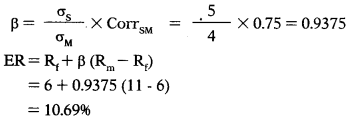Question 62.
Zebra Ltd. has a beta (P) of 1.15. The return on market portfolio is 1496. The risk¬free rate of return is 596. Actual rates of returns over 4 observations are as under: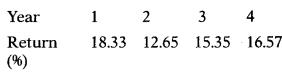In which year security is undervalued.
(A) Year 1& Year 3
(B) Year 2 & Year 3
(C) Year 3 & Year 4
(D) Year 1 & Year 4
(D) Year 1 & Year 4
ER=Rf+p(Rm-Rf)
= 5+1.15(14-5)
= 15.35%Question 63.
Return on Lucky Ltd.’s shares has a standard deviation of 2296, as against the standard deviation of the market at 1296. Correlation co-efficient between market and stock of Lucky Ltd. is 0.796. Compute unsystematic risk of Lucky Ltd.’s shares.
(A) 15.496
(B) 8.496
(C) 6.696
(D) 12.696
(C) 6.696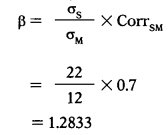Systematic risk = Beta × SD of market
= β×σM = 1.2833 × 12 = 15.4%
Unsystematic risk = Total risk – Systematic risk
= 22 – 15.4
= 6.696

Question 64.
In a portfolio of the company,₹ 2,00,000 have been invested in Asset-X which has an expected return of 8.596, ₹ 2,80,000 in Asset-Y, which has an expected return of 10.296 and ₹ 3,20,000 in Asset-Z which has an expected return of 1296. What is the expected return for the portfolio?
(A) 12.94596
(B) 10.49596
(C) 10.54996
(D) 15.94596
(B) 10.49596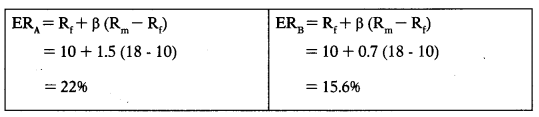Question 65.
The following data relate to two securities, A and B: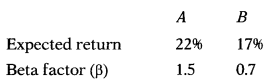Assume RF = 10% and RM = 18%.
Find out whether the securities, A and B are correctly priced?
Securities A — Securities B
(A) Undervalued — Overvalued
(B) Overvalued — Undervalued
(C) Correctly valued — Undervalued
(D) Overvalued — Correctly valued